Courses

# Test: Fractions And Decimals - 1

## 30 Questions MCQ Test Mathematics (Maths) Class 7 | Test: Fractions And Decimals - 1

Description
This mock test of Test: Fractions And Decimals - 1 for Class 7 helps you for every Class 7 entrance exam. This contains 30 Multiple Choice Questions for Class 7 Test: Fractions And Decimals - 1 (mcq) to study with solutions a complete question bank. The solved questions answers in this Test: Fractions And Decimals - 1 quiz give you a good mix of easy questions and tough questions. Class 7 students definitely take this Test: Fractions And Decimals - 1 exercise for a better result in the exam. You can find other Test: Fractions And Decimals - 1 extra questions, long questions & short questions for Class 7 on EduRev as well by searching above.
QUESTION: 1

### What is the fraction of the shaded area?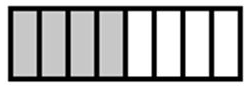Solution:

Total number of blocks = 8

Number of shaded blocks = 4

Number of unshaded blocks = 4

Fraction of Shaded area =  No. of blocks of shaded area / Total number of blocks

4 / 8 = 1/2

So option A is the correct answer.

QUESTION: 2

### What is the fraction of the shaded area?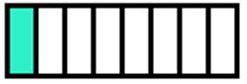Solution:

Total number of blocks = 8

Number of shaded blocks = 1

Number of unshaded blocks = 7

Fraction of Shaded area =  No. of shaded blocks/ Total number of blocks

= 1 / 8

So option B is the correct answer.

QUESTION: 3

### What is the fraction of the shaded area?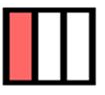Solution:

Total number of blocks = 3

Number of shaded blocks = 1

Number of unshaded blocks = 2

Fraction of Shaded area =  No. of shaded blocks/ Total number of blocks

= 1 / 3

So option B is the correct answer.

QUESTION: 4

What is the fraction of the shaded area?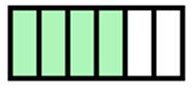Solution:

Total number of blocks = 6

Number of shaded blocks = 4

Number of unshaded blocks = 2

Fraction of Shaded area =  No. of shaded blocks/ Total number of blocks

= 4 / 6 = 2/3

So option A is the correct answer.

QUESTION: 5

What is the fraction of the shaded area ?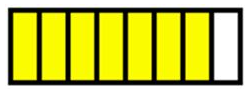Solution:

Total number of blocks = 8

Number of shaded blocks = 7

Number of unshaded blocks = 1

Fraction of Shaded area =  No. of shaded blocks/ Total number of blocks

=7/8

So option C is the correct answer.

QUESTION: 6

When 0.232323..... is converted into a fraction, then the result is:

Solution:

0.23232323…=0.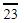Let x=0.Multiplying both sides by 100
100x=23.Subtracting
100x-x=23.-0.99x=23
x=23/99

QUESTION: 7

Thrice the first of three consecutive odd integers is 3 more than twice the third. The third integer is:

Solution:

Let the three integers be xx + 2 and x + 4.

Then, 3x = 2(x + 4) + 3  ⇔  x = 11.

∴ Third integer = x + 4 = 15.

QUESTION: 8

(1/2)x(1/5)= ____

Solution:

(1/2)x(1/5) = 1 / 10

So option B is correct answer.

QUESTION: 9

If 2994 ÷ 14.5 = 172, then 29.94 ÷ 1.45 = ?

Solution:

Since 2994 is divided by 100 and 14.5 is divided by 10 that is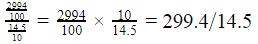So there is only one decimal shift that is why answer also has one decimal shift which is 17.2

QUESTION: 10

0.01 × 0.01 = ______

Solution:

0.01 × 0.01 = 0.0001

So option A is the correct answer.

QUESTION: 11

Arrange the following in descending order:

(2/9), (2/3), (8/21)

Solution:

Given :

(2/9), (2/3), (8/21)

First we convert each fraction into like fraction.

2/9 = (2 x 7)/(9 x 7) = 14/63.

2/3 = (2 x 21)/(3 x 21) = 42/63.

8/21 = (8 x 2)/(21 x 3) = 24/63.

If denominators are same, then, greater the numerator, greater will be the fraction.

Here, 42 > 24 > 14

So the fractions in descending order will be:(2/3) > (8/21) > (2/9).

So option A is the correct answer.

QUESTION: 12

Ritu ate 3/5 part of an apple and the remaining apple was eaten by her brother Somu. How much part of the apple did Somu eat?

Solution:

The part of the apple eaten by Ritu = 3/5

The part of the apple eaten by Somu = 1 - (3/5) = (5 - 3)/5 = 2/5.

QUESTION: 13

One side of a square is 2/a, its perimeter is

Solution:

We know, Perimeter of a square is 4a

Given, a = 2/a

So perimeter = 4a = 4 x 2/a = 8/a

So option D is the correct answer.

QUESTION: 14

What will be  the average of  0.3, 3, 0.03 , and 0.002

Solution:

Given numbers are :

0.3 , 3 , 0.03 and 0.002

We know that Average =  Sum of numbers divided by total numbers

Average = 0.3 + 3 + 0.03 + 0.002 / 4

= 3.332 / 4

= 0.833

So, Average = 0.833

Therefore, option A is the correct answer.

QUESTION: 15

The result of adding the difference of 3.003 and 2.05 to their sum is

Solution:

Given numbers are :

3.003 and 2.05

Now, Sum of numbers = 3.003 + 2.05

= 5.053

Difference of numbers = 3.003 - 2.05

= 0.953

Adding the Difference of numbers to the Sum of numbers , we get

5.053 + 0.953 = 6.006

So option A is the correct answer.

QUESTION: 16

Find the area of rectangle whose length is 6.7 cm and breadth is 2 cm.

Solution:

Given ,

Length =  6.7 cm

Area of rectangle = L X B

= (6.7 x 2 ) cm

= 13.4 cm2

So option B is the correct answer.

QUESTION: 17

If 43m =0.086 then m has the value

Solution:

43m = 0.086

m = 0.086 / 43

m = 0.002

So option A is the correct answer.

QUESTION: 18

The value of  2.5 × 0.3 is :

Solution:

2.5 × 0.3 = 0.75

So option A is the correct answer.

QUESTION: 19

The fraction (p+q)/q equals

Solution:

The fraction ( p+q)/q = p/q + q/q

i.e, p/q + 1

So option C is the correct answer.

QUESTION: 20

What is 3/5 of 25

Solution:

Explanation :

= 3/5 x 25 = 3 x 5

= 15

So option C is the correct answer.

QUESTION: 21

5/7 ÷ 1/2 is equal to

Solution:

= 5/7 ÷ 1/2

=  5/7 x 2/1

=  10 / 7

So option C is the correct answer.

QUESTION: 22

Which of the following is an improper fraction?

Solution:

A fraction in which the numerator is greater than the denominator is improper fraction.

Since in the given fraction 50 / 20

50 is greater than 20. So it is an improper fraction .

So option C is the correct answer.

QUESTION: 23

Which of the following is an equivalent fraction of  3/10?

Solution:

Two fractions are said to be equivalent fractions if the simplest form of both the fractions is the same. So 6/20=3/10 , 5/20=¼ and 1/40 =1/40 .So equivalent fraction of 3/10 is 6/20

QUESTION: 24

2 / 3 of 66 is:

Solution:

Given 2 / 3 of 66 = 2 / 3 x 66 = 44

Hence , 2/3 of 66 is 44

So option D is the correct answer.

QUESTION: 25

The product of two proper fractions is ........ than each of the fractions that are multiplied

Solution:

The product of two proper fractions is less than each of the fractions that are multiplied .

e.g, 1 /2 x 1 / 4 = 1 / 8

1 / 8 < 1 / 2 and 1 / 8 < 1 / 4.

QUESTION: 26

Which two fractions are equivalent?

Solution:

divide whole of the fraction 2/4 by 2, we get 1/2.

QUESTION: 27

Express as rupees using decimals: 7 rupees 7 paise

Solution:

Rs.7 + Rs 7 / 100 + Rs  0.07 = Rs 7.07

QUESTION: 28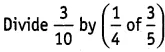Solution: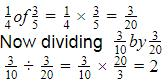QUESTION: 29

2.05 x 1.3 equals to

Solution:

When you multiply 2.05 by 1.3 you get 2.665 , because there are three decimal places.

QUESTION: 30

What will be the value of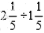Solution: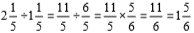= 11/6

So option C is the correct answer.